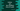# Python program to get the last modified time of a path## Get the last modified time of a path in python:

To get the last modified time of a path, we can use the inbuilt os module. Python os module provides different operating system related methods. os.path is a submodule of os. It has few useful path related functions.

os.path submodule has one method called getmtime that returns the last modified time for a given path.

In this post, we will learn how to use getmtime method with example.

### Definition of getmtime:

getmtime is defined as below:

``os.path.getmtime(path)``

Here,

• path is the give path. It can be a path of a file, folder etc.
• It returns a floating point value. This is the last modified time for that given path. This floating point value is actually the number of seconds since epoch. If the file doesn’t exists or if it is inaccessible, it throws a OSError.

Once we get the last modified value, we can use any time package to convert it to a human readable format.

### Example of getmtime:

Let’s take a look at the below program:

``````import os

Here, given_path is the path of a file for which we are finding the last modified time. If you run this program, it will give output something like below:

``Last modified time: 1616219535.8482218``

This is not in a human readable format. Let’s try to make it more easy to read.

### Python program to get the last modified time in human readable format:

The return value of os.path.getmtime is a floating point value which is the number of seconds since epoch time. Most python datetime library handles this time and we can use any library to convert it to a human readable format.

Let’s use the datetime module. This is an inbuilt module and we don’t have to install any third party library to use it. Just use import datetime.

datetime module provides one method called fromtimestamp which takes the epoch time as its parameter and returns the date-time in human readable format.

The below program uses datetime module to print the last modified time in human readable format:

``````import os
import datetime

last_modified = os.path.getmtime(given_path)
last_modified_time = datetime.datetime.fromtimestamp(last_modified)

Here,

• We are using the same path as the previous example.
• The last modified epoch time is calculated and stored in the last_modified variable.
• lastmodifiedtime variable converts this value to human readable format.

The last print statement prints this value.

It will print output as like below:

``Last modified time: 2021-03-20 11:22:15.848222``